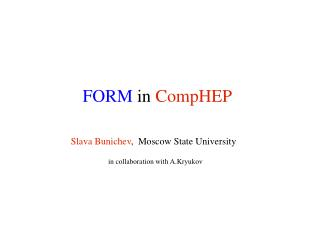# FORM in CompHEP - PowerPoint PPT PresentationDownload PresentationFORM in CompHEP

FORM in CompHEP
Download Presentation## FORM in CompHEP

- - - - - - - - - - - - - - - - - - - - - - - - - - - E N D - - - - - - - - - - - - - - - - - - - - - - - - - - -
##### Presentation Transcript

1. FORMinCompHEP Slava Bunichev, Moscow State University in collaboration with A.Kryukov

2. CompHEP • CompHEP is a package for computation of Feynman diagram in quantum field theory from Lagrangian until event flow. • Main feature of the package is an exact symbolic calculation of tree level diagrams for Standard Model and beyond (2HDM, MSSM, mSUGRA, ... ). • CompHEP is able to compute processes with many particles (ap to 6) in the finale state taking into account all the QCD and EW diagrams, masses of fermions and bosons and width of unstable particles. • CompHEP has user-friendly GUI and highly optimized for fast calculations and effective memory usage.

3. Price for this approach was high specialization of the module for the symbolic calculations. For example, a fixed number of symbolic structures allows in symbolic expressions under evaluation. Such specialization is too heavy for further development of CompHEP package!

4. The symbolic part includes diagram generator and symbolic calculator. The numerical part includes Monte Carlo integration module and event generator. We have to change the module for symbolic calculations. CompHEP structure:

5. To have more advantage symbolic part of CompHEP we incorporate the computer algebra FORM for automatic evaluation of squared Feynman diagrams. We choose the FORM because: FORM is rather fast and good quality computer algebra program, FORM is a standard de-facto in HEP applications. FORM

6. To save the compatibility of old version of CompHEP with new one and to minimize programming we decide to incorporate FORM in a parallel. Thus, the new scheme was realized by adding the following modules: “form_code.c” is the FORM code generator “procedur.prc” is a set of auxiliary FORM programs CompHEP-FORM-CompHEP

7. Scheme of the CompHEP-FORM-CompHEPinterface

8. e+,e-+,- Example of diagrams generating

9. Example of symbolic calculations

10. Example of numerical calculations

11. #include<math.h> #define real double #include"out_ext.h" #include"out_int.h" #include"var_def.h" FNN F1; real F1(void) { real FACTOR,RNUM,DENOM,result; FACTOR= 1./4.*pow(EE,4); RNUM= (32*pow(Mm,2) + 32*dp(0) - 64*dp(1))*dp(0) + 64*pow(dp(1),2); DENOM= 4*pow(dp(0),2); result=FACTOR*RNUM/DENOM; return result; } Example of the FORM output

12. We have to optimize C-code for improving calculation time in numerical part. So we introduce the algorithm for reducing number of multiplications in algebraic expressions. (results of working this algorithm is similar with Gorner scheme) Optimization

13. 3*d1*d2*d3 + 5*d22*d3 + 7*d2*d32 + 2*d1*d3 + 3*d2 = rename: d1=d11, d12=d11*d12, ... 3*d11*d21*d31 + 5*d21*d22*d31 + 7*d21*d31*d32 + 2*d11*d31 + 3*d21 = find most rare multiplier: 3*d11*d21*d31 + 5*d21*d22*d31 + 7*d21*d31*(d32) + 2*d11*d31 + 3*d21 = 3*d11*d21*d31 + 5*d21*(d22)*d31 + 7*d21*d31*(d32) + 2*d11*d31 + 3*d21 = combine terms with similar terms: 3*d11*d21*d31 + (5*d22 + 7*d32)*d21*d31 + 2*d11*d31 + 3*d21 = repeat procedure: 3*(d11)*d21*d31 + (5*d22 + 7*d32)*d21*d31 + 2*(d11)*d31 + 3*d21 = (3*d11 + 5*d22 + 7*d32)*d21*d31 + 2*(d11)*d31 + 3*d21 = (3*d11 + 5*d22 + 7*d32)*(d21)*d31 + 2*(d11)*d31 + 3*(d21) = ((3*d11 + 5*d22 + 7*d32)*d21 + 2*d11)*d31 + 3*(d21) = ((3*d1 + 5*d2 + 7*d3)*d2 + 2*d1)*d3 + 3*d2 Bu-algorithm

14. Conclusion • We completely incorporate FORM in CompHEP • Crosscheck (we get the same results for both calculators) • We optimize FORM-output for numerical calculation

15. Future plans: • We plan to implement new algorithms to increase efficiency of symbolic calculations • Perform calculations in theories with extra dimensions • Introduce new complicated structures in the vertices (e.g. Form-factors) • Use the dimentional regularization • Perform polarized calculations by introducing the corresponding density matrices for external lines of squared diagrams. • Include calculations with extention to 1-loop case • Incorporate the gauge invariant classes of diagrams, etc.